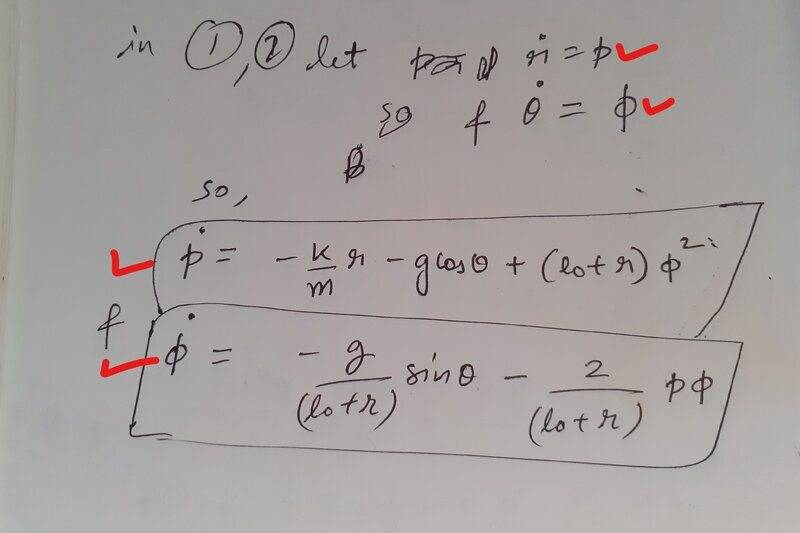# Spring Pendulum system in FORTRAN

• Fortran
• Abhisheko07
In summary, the programmer is having trouble getting the program to accept the input values. One of the variables approaches zero, and the programmer doesn't know why.f

#### Abhisheko07

TL;DR Summary
Can anyone help me figure out what is going wrong?
I have checked the program several times the program is running but the graphs I am getting is not what I was accepting soon one of the variables approaches zero I don't know why is it happening. The program I have made is below and it's in the FORTRAN language. If anyone knows Fortran can they please help me it's really very important for my project work?

Fortran:
                            implicit real*8(a-h,o-z)
real*8 m,l
ti=0
tf=1000
dt=0.01
n=(tf-ti)/dt

! initial values
t1=0.5
r=2.0
td=0.3
p=1.0
l=3.0
m=2.0
t=ti

open(1,file='elast1.dat',status='unknown')
open(2,file='elast2.dat',status='unknown')
open(3,file='elast3.dat',status='unknown')

!starting the loop
do i=1,n
write(1,*)t,t1,td
write(2,*)t,r,p
write(3,*)t,t1,r

fa=f(t1,r,td,p,m,l)*dt
f1a=f1(td)*dt

ea=e(t1,r,td,p,m,l)*dt
e1a=e1(p)*dt

fb=f(t1+0.5*f1a,r+0.5*e1a,td+0.5*fa,p+0.5*ea,m,l)*dt
f1b=f1(td+0.5*fa)*dt

eb=e(t1+0.5*f1a,r+0.5*e1a,td+0.5*fa,p+0.5*ea,m,l)*dt
e1b=e1(p+0.5*ea)*dt

fc=f(t1+0.5*f1b,r+0.5*e1b,td+0.5*fb,p+0.5*eb,m,l)*dt
f1c=f1(td+0.5*fb)*dt

ec=e(t1+0.5*f1b,r+0.5*e1b,td+0.5*fb,p+0.5*eb,m,l)*dt
e1c=e1(p+0.5*eb)*dt

fd=f(t1+f1c,r+e1c,td+fc,p+ec,m,l)*dt
f1d=f1(td+fc)*dt

ed=f(t1+f1c,r+e1c,td+fc,p+ec,m,l)*dt
e1d=e1(p+ec)*dt

td=td+(fa/6.0)+(fb/3.0)+(fc/3.0)+(fd/6.0)
p=p+(ea/6.0)+(eb/3.0)+(ec/3.0)+(ed/6.0)

t1=t1+(f1a/6.0)+(f1b/3.0)+(f1c/3.0)+(f1d/6.0)
r=r+(e1a/6.0)+(e1b/3.0)+(e1c/3.0)+(e1d/6.0)

t=t+dt

enddo
close(1)
close(2)
end

!Function (definition of theta double dot)
function f(t1,r,td,p,m,l)
implicit real*8(a-h,o-z)
real*8 m,l

g=9.8
xa=-(g*dsin(t1))/(l+r)
xb=-(2*p*td)/(l+r)
f=xa+xb

return
end

!Function (definition of theta dot)
function f1(td)
implicit real*8(a-h,o-z)
f1=td

return
end

! Function (definition of r double dot)
function e(t1,r,td,p,m,l)
implicit real*8(a-h,o-z)
real*8 m,l
g=9.8
k=10.0
xc=-(k*r)/m
xd=g*dcos(t1)
xe=(l+r)*td**2
e=cx+xd+xe

return
end

! Function (definition of r dot)
function e1(p)
implicit real*8(a-h,o-z)
e1=p
end

These are the four equations I have used (actually they are two 2nd order ordinary differential equation but i have to convert it into four equations to do the programming)Last edited by a moderator:
Shouldn’t the sign of xd in function e be Negative?

Get rid of the implicit none!

On line 97, you mistyped xc as cx.

Implicit none is archaic and kept inly for backwards compatibility. Get into the habit of using strong typing. You will thank me later for it!

••Abhisheko07, jedishrfu and Paul Colby
Implicit none is archaic and kept only for backwards compatibility.
Um, implicit none is what you need to give you strong typing.

•DrClaude
Shouldn’t the sign of xd in function e be Negative?
no actually there was a mistake the sign in the program is all ok but the picture sign was incorrect and finally after reviewing it again i got the mistake which is that in place of xc i used cx very silly but vital mistake.

thanks a lot it worked

i got the mistake which is that in place of xc i used cx very silly but vital mistake.
This is a learning moment. As others have pointed out using,” implicit none“ would have caught this mistype right away.

Um, implicit none is what you need to give you strong typing.Oh my, what a brain fart! Of course, what I mean is to get rid of the implicit and replace it by implicit none.

On line 97, you mistyped xc as cx.
The two main problems are see are 1) the use of mostly meaningless names for variables and functions, and 2) the near-complete lack of comments.

All of the variable names in this program are one or two letters in length, so they don't give any clue to outside readers what they are being used for. Using extremely short variable names makes mistakes like switching xc to cx much more likely.

I count a grand total of four comments, all for the functions defined near the bottom of the code.
f -- theta double dot
f1 -- theta dot
e -- r double dot
e1 -- r dot
The comments are somewhat helpful, but a better choice of function names would have made it clearer what they're being used for. e, e1, f, and f1, especially at the point at which these functions are being called. Also, some of these functions take multiple parameters, of which there are exactly zero comments as to what the parameters represent.

•DrClaude